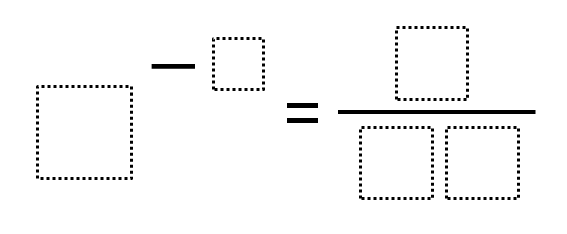Home > Grade 8 > Expressions & Equations > Negative Exponents – Closest to Zero

# Negative Exponents – Closest to Zero

Directions: Use the digits 0 to 9, at most one time each, to fill in the boxes to to make a result that is as close to zero as possible.### Hint

What happens when you have a negative exponent?
Where does the 1 go?

So far, there are two answers that have a result of 1/64.

8 ^ (-2) = 1 / 64
4 ^ (-3) = 1 / 64

Source: Daniel Luevanos

## Minimize Slope

Directions: Given the point (3,5), use digits 1-9, at most one time, to find a …

1.•2.